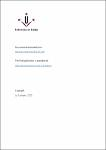## Highest weak focus order for trigonometric Liénard equations2020
Gasull, Armengol
Valls, Claudia
##### Abstract
Given a planar analytic differential equation with a critical point which is a weak focus of order k, it is well known that at most k limit cycles can bifurcate from it. Moreover, in case of analytic Liénard differential equations this order can be computed as one half of the multiplicity of an associated planar analytic map. By using this approach, we can give an upper bound of the maximum order of the weak focus of pure trigonometric Liénard equations only in terms of the degrees of the involved trigonometric polynomials. Our result extends to this trigonometric Liénard case a similar result known for polynomial Liénard equations.
##### Journal or Serie
Annali di Matematica Pura ed Applicata, 2020, vol.199, p. 1673-1684